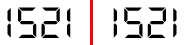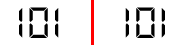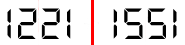Problem Statement

A number is written on a traditional digital display. If the display looks identical when flipped horizontally (i.e., around a vertical axis), the number is called a "mirror number". On a digital display, the digits 0, 1 and 8 are symmetrical, and the digits 2 and 5 are mirror images of each other. No other digits make sense when flipped horizontally. For example, 0, 101 and 1521 are all mirror numbers, while 1221 and 1010 are not (see images below). Given two Strings A and B, both representing integers with no extra leading zeroes, return the number of mirror numbers between A and B, inclusive.

Mirror numbers (both remain unchanged after mirroring):Not mirror numbers (1221 mirrors to 1551, and 1010 mirrors to 0101):Definition

 Class: MirrorNumber Method: count Parameters: String, String Returns: int Method signature: int count(String A, String B) (be sure your method is public)

Constraints

-A will represent an integer between 0 and 10^18, inclusive.
-B will represent an integer between A and 10^18, inclusive.
-Both A and B will have no extra leading zeros.

Examples

0)

 "0" "10"
Returns: 3
 There is only 0, 1 and 8 here.
1)

 "0" "100"
Returns: 7
 Few more: 11, 25, 52, 88.
2)

 "143" "23543"
Returns: 54

Problem url:

http://www.topcoder.com/stat?c=problem_statement&pm=7869

Problem stats url:

http://www.topcoder.com/tc?module=ProblemDetail&rd=10777&pm=7869

mateuszek

Testers:

PabloGilberto , Olexiy , lovro , ivan_metelsky

Problem categories:

Recursion, Search, Simple Math